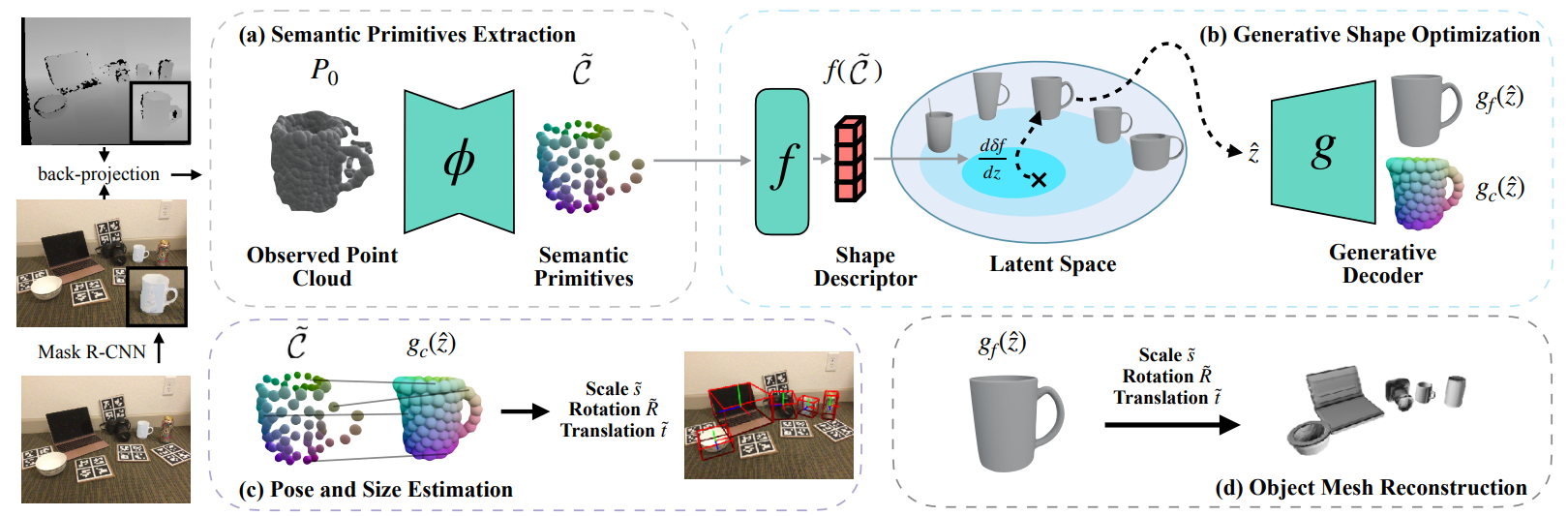# CoRL 2022

1State Key Lab of CAD & CG, Zhejiang University 2ByteDance AI Lab
3Multimedia Laboratory, The Chinese University of Hong Kong

## Abstract

Empowering autonomous agents with 3D understanding for daily objects is a grand challenge in robotics applications. When exploring in an unknown environment, existing methods for object pose estimation are still not satisfactory due to the diversity of object shapes. In this paper, we propose a novel framework for category-level object shape and pose estimation from a single RGB-D image. To handle the intra-category variation, we adopt a semantic primitive representation that encodes diverse shapes into a unified latent space, which is the key to establish reliable correspondences between observed point clouds and estimated shapes. Then, by using a SIM(3)-invariant shape descriptor, we gracefully decouple the shape and pose of an object, thus supporting latent shape optimization of target objects in arbitrary poses. Extensive experiments show that the proposed method achieves SOTA pose estimation performance and better generalization in the real-world dataset.

## Pipeline OverviewOverview of the proposed method. The input of our method is the point cloud observed from a single-view depth image. (a) Our method first extracts semantic primitives $\tilde{\mathcal{C}}$ from object point cloud $P_0$ by a part segmentation network $\phi$. (b) We calculate a SIM(3)-invariant shape descriptor $f(\tilde{\mathcal{C}})$ from $\tilde{\mathcal{C}}$ and optimize a shape embedding $\hat{z}$ in the latent space by the difference between $f(\tilde{\mathcal{C}})$ and $f(g_c(z))$, where $g_c$ and $g_f$ are the coarse and fine branches of the generative model $g$, detailed in Sec. 3.1. (c) The similarity transformation $\{\tilde{s},\tilde{\boldsymbol{R}},\tilde{\boldsymbol{t}}\}$ is recovered through the semantic correspondences between $\tilde{\mathcal{C}}$ and optimized shape $g_c(\hat{z})$. (d) Further, we can simply apply the transformation $\{\tilde{s},\tilde{\boldsymbol{R}},\tilde{\boldsymbol{t}}\}$ on the fine geometry generated through $g_f(\hat{z})$, and reconstruct the object-level scene as a by-product besides our main results, which is detailed in the Sec. B of the appendix.

## Visualization of Shape Optimization

Visualization of our shape optimization process. The objects in the figure are optimized by 20 iterations (from left to right). As the optimization proceeds, the shape of the objects changes significantly. For example, the lens of the camera is gradually stretched, the angle between the screen and keyboard of the laptop gradually increases, and the handle of the mug becomes rectangular.

## BibTeX

@inproceedings{
li2022generative,
title={Generative Category-Level Shape and Pose Estimation with Semantic Primitives},
author={Li, Guanglin and Li, Yifeng and Ye, Zhichao and Zhang, Qihang and Kong, Tao and Cui, Zhaopeng and Zhang, Guofeng},
booktitle={6th Annual Conference on Robot Learning},
year={2022},
url={https://openreview.net/forum?id=N78I92JIqOJ}
}